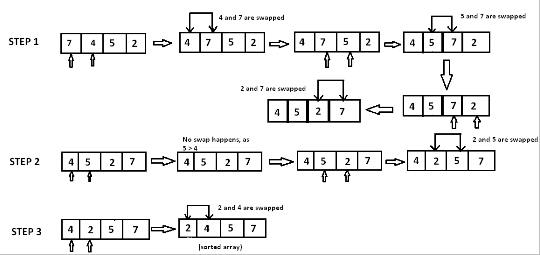# Python Program for Bubble Sort

The figure shown below illustrates the working of this algorithm −## Approach

• Starting with the first element(index = 0), compare the current element with the next element of the array.

• If the current element is greater than the next element of the array, swap them.

• If the current element is less than the next element, move to the next element.

Repeat Step 1.

Now let’s see the implementation below −

## Example

def bubbleSort(ar):
n = len(arr)
# Traverse through all array elements
for i in range(n):
# Last i elements are already in correct position
for j in range(0, n-i-1):
# Swap if the element found is greater than the next element
if ar[j] > ar[j+1] :
ar[j], ar[j+1] = ar[j+1], ar[j]
# Driver code to test above
ar = ['t','u','t','o','r','i','a','l']
bubbleSort(ar)
print ("Sorted array is:")
for i in range(len(ar)):
print (ar[i])

## Output

Sorted array is:
a
i
o
r
t
t
u
l

## Conclusion

In this article, we learnt about the approach to do Bubble sort in Python 3.x. Or earlier